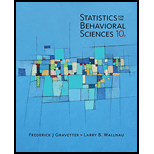# a. After 6 points have been added to every score in a sample the mean is found to be M = 10 and the standard deviation is s = 13. What were the values for the mean and standard deviation for the original sample? b. After every score in a sample is multiplied by 3, the mean is found to be M = 48 and the standard deviation is .f = l 8 what were the values for the mean and standard deviation for the original sample?### Statistics for The Behavioral Scie...

10th Edition
Frederick J Gravetter + 1 other
Publisher: Cengage Learning
ISBN: 9781305504912### Statistics for The Behavioral Scie...

10th Edition
Frederick J Gravetter + 1 other
Publisher: Cengage Learning
ISBN: 9781305504912

#### Solutions

Chapter
Section
Chapter 4, Problem 15P
Textbook Problem

## Expert Solution

### Want to see the full answer?

Check out a sample textbook solution.See solution

### Want to see this answer and more?

Experts are waiting 24/7 to provide step-by-step solutions in as fast as 30 minutes!*

See Solution

*Response times vary by subject and question complexity. Median response time is 34 minutes and may be longer for new subjects.

Find more solutions based on key concepts
Show solutions
Evaluate the expression sin Exercises 116. 33

Finite Mathematics and Applied Calculus (MindTap Course List)

Express the function in the form f g. 48. u(t)=tant1+tant

Single Variable Calculus: Early Transcendentals, Volume I

Fill in the blanks. 6. The statement limxa f(x) = L means that the values of __________ can be made as close to...

Applied Calculus for the Managerial, Life, and Social Sciences: A Brief Approach

Find the total current in each parallel circuit:

Elementary Technical Mathematics

If 90A180, then A/2 terminates in which quadrant?

Trigonometry (MindTap Course List)

Find dy/dx by implicit differentiation. 5. x2 4xy + y2 = 4

Single Variable Calculus: Early Transcendentals

True or False: is a convergent series.

Study Guide for Stewart's Multivariable Calculus, 8th

True or False: If f(x) 0 for all x in an interval I then f is increasing on I.

Study Guide for Stewart's Single Variable Calculus: Early Transcendentals, 8th

M&M Candy Colors. Mars, Inc. manufactures M&M’s, one of the most popular candy treats in the world. The milk ch...

Modern Business Statistics with Microsoft Office Excel (with XLSTAT Education Edition Printed Access Card) (MindTap Course List)

Estimating Rates of Change with the CalculatorMake a graph of x3x2 and use the calculator to estimate its rate ...

Functions and Change: A Modeling Approach to College Algebra (MindTap Course List)

Rocket Motion Suppose a small single-stage rocket of total mass m(t) is launched vertically, the positive direc...

A First Course in Differential Equations with Modeling Applications (MindTap Course List)